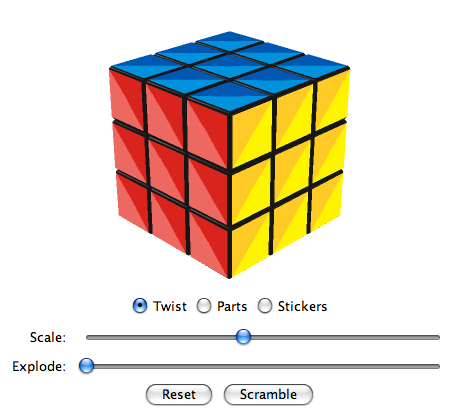# Virtual CubesActivate JavaScript to see the Virtual Cubes!

### Triangular Super Cube

A Super Cube is a design variation of the Rubik's Cube which visualizes the orientation of side parts. Because of this, a Super Cube has 2048 times more positions than a regular cube (46/2 = 2048).

Compared to a Rubik's Cube, the stickers are divided into two triangles where one of them is brighter then the other.

The layout of the Triangular Super Cube was created in 2010 by Ortwin Schenker. The design was inspired by Georges Helm's Triangle Cube.

#### Algorithms to twist the centers

Algorithm
(U R U R')5 (20 ltm, 20 ftm, 20 qtm)
Permutation (++u)

Algorithm M. B. Thistlethwaite
(U · R L · U2 · R' L')2 (12* ltm, 12* ftm, 14 qtm)
Permutation (++u)

Algorithm
(U' R2 U2 R' U2 R2)3 (18 ltm, 18 ftm, 30 qtm)
Permutation (+u) (+r)

Algorithm Herbert Kociemba 2008
D' R · U D · R2 U2 MF MD B MD' MF' U2 R2 U' (14* ltm, 18 ftm, 22 qtm)
Permutation (+u) (+r)

Algorithm
(MR' MD' MR) U' (MR' MD MR) U (8* ltm, 14* ftm, 14* qtm)
Permutation (+u) (-r)

Algorithm
(MR' MD' MR) U2 (MR' MD MR) U2 (8* ltm, 14 ftm, 16* qtm)
Permutation (++u) (++r)

Algorithm
(MR' MD2 MR) U' (MR' MD2 MR) U (8* ltm, 14* ftm, 18* qtm)
Permutation (+u) (-d)

Algorithm
(MR' MD2 MR U2)2 (8* ltm, 14 ftm, 20 qtm)
Permutation (++u) (++d)

Algorithm Herbert Kociemba 2008
CD' · MR MD MR' D MR MF MR' F' MD MF' MD' F U' (13* ltm, 22 ftm, 22* qtm)
Permutation (+u) (+r) (+f) (+d) (-l) (+b)

Notation